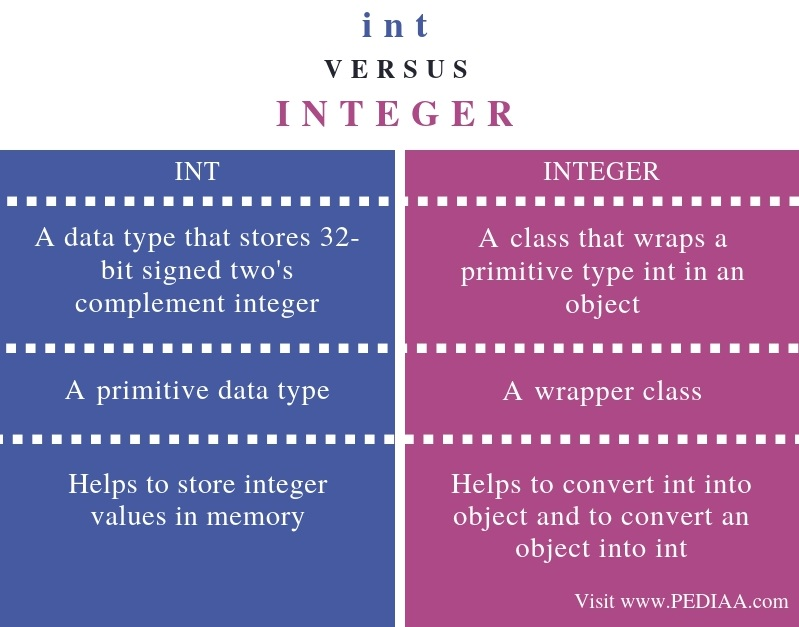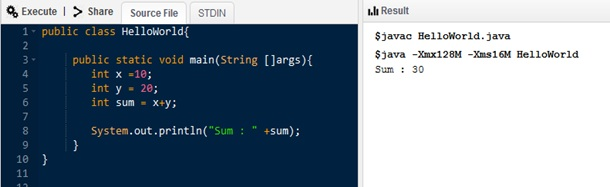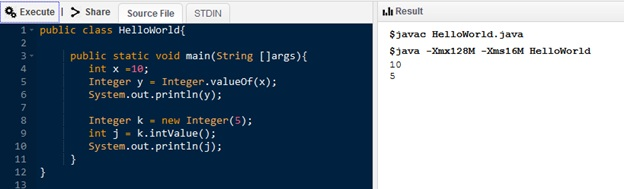# What is the Difference Between int and Integer in Java

The main difference between int and Integer in Java is that an int is a primitive data type while an Integer is the wrapper class corresponding to int.

Java is a powerful programming language to develop secure and robust applications. One major reason for the popularity of Java is that it supports Object Oriented Programming. Further, this paradigm allows modeling real-world scenarios easily to develop the software. Also, the common use of this language is in the web, mobile and other enterprise level applications. Two concepts related to Java are int and Integer.

### Key Areas Covered

1. What is int in Java
– Definition, Functionality
2. What is Integer in Java
– Definition, Functionality
3. What is the Difference Between int and Integer in Java
– Comparison of Key Differences

### Key Terms

int, Integer, OOP## What is int

A variable is a memory location that holds a value. Each variable has a datatype. In other words, each variable is capable of storing data of a specific type. The data type defines how much memory to allocate for storing a value at that memory location.

One main data type in Java is a primitive data type; these are the basic data types available in Java.  ‘int’ is one of them. The default value of an int variable is 0. Moreover, it allocates 4 bytes when storing data.

An example is as follows.Figure 1: Java program with int

In the above program, x and y are two variables of type int. They store value 10 and 20. There is another int type variable called sum.  The total of x and y is stored in the sum variable. Finally, the println method displays the output on the console.

## What is Integer

Wrapper classes help to convert a primitive data type to an object and object to a primitive type. Data structures such as lists, vectors, etc. do not store data in primitive format. They store data as objects. Therefore, we can use wrapper classes to perform this conversion. The equivalent wrapper class for int is Integer.Figure 2: Java program with Integer

In the above program, x is an int value. We can convert that to an Integer like in line 5. Then the println method displays that output on the console. Similarly, k is an Integer. We can convert that to an int using the intValue method. Finally, the println method displays the output on the console.

## Difference Between int and Integer in Java

### Definition

While int is a data type that stores 32-bit signed two’s complement integer, an integer is a class that wraps a primitive type int in an object.

### Basis

Thus, the main difference between int and Integer in Java is that int is a primitive data type while Integer is a wrapper class.

### Usage

While int data type helps to store integer values in memory, integer helps to convert int into an object and to convert an object into an int. Hence, this is another difference between int and Integer in Java.

### Conclusion

The main difference between int and Integer in Java is that an int is a primitive data type while Integer is the wrapper class corresponding to int. In brief, we can use int to store values such as 5,10, 20, etc. On the other hand, we can use Integer to convert an int to an object and to convert an object to an int.

##### Reference:

1. “Java Data Types – Javatpoint.” Www.javatpoint.com, Available here.
2. “Wrapper Class in Java – Javatpoint.” Www.javatpoint.com, Available here.
3. “What Every Computer Scientist Should Know About Floating-Point Arithmetic”, 6 Oct. 2018, Available here.